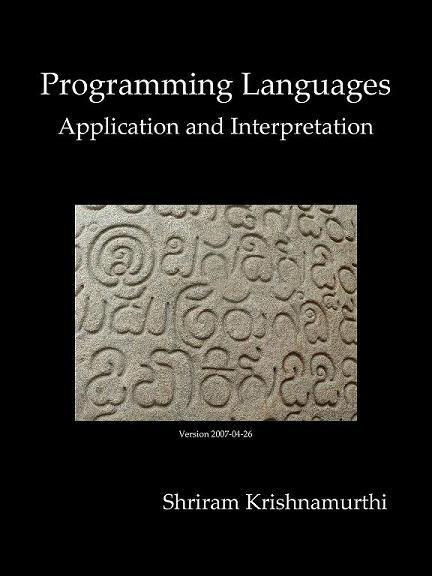GitHub：PLAI-cn

GitBook：PLAI-cn

## 1.3 本书使用的语言

``````#!/bin/sh
``````

``````<!DOCTYPE HTML PUBLIC "-//W3C//DTD HTML 4.01//EN" ...>
``````

``````plai-typed
``````

``````#lang plai-typed
``````

Typed PLAI语言和传统Racket最主要的不同是它是静态类型的。它还给你提供了些有用的的东西（construct）：`define-type``type-case``test`。【注释】下面是它们的使用实例。创建新的数据类型：

``````(define-type MisspelledAnimal
[caml (humps : number)]
[yacc (height : number)])
``````

``````(caml 2)
(yacc 1.9)
``````

``````(define ma1 : MisspelledAnimal (caml 2))
(define ma2 : MisspelledAnimal (yacc 1.9))
``````

``````(define ma1 (caml 2))
(define ma2 (yacc 1.9))
``````

``````(define (good? [ma : MisspelledAnimal]) : boolean
(type-case MisspelledAnimal ma
[caml (humps) (>= humps 2)]
[yacc (height) (> height 2.1)]))
``````

``````(test (good? ma1) #t)
(test (good? ma2) #f)
``````

``````(define (good? [ma : MisspelledAnimal]) : boolean
(type-case MisspelledAnimal ma
[caml (h) (>= h 2)]
[yacc (h) (> h 2.1)]))
``````

``````(define (good? [ma : MisspelledAnimal]) : boolean
(cond
[(caml? ma) (>= caml-humps ma) 2]
[(yacc? ma) (> (yacc-height ma) 2.1)]))
``````

## 2 本书有关语法解析的一切

``````23 + 5 - 6
``````

## 2.1 轻量级的，内建的语法解析器的前半部分

``````(+ 23 (- 5 6))
``````

——会产出一列表，其第一个元素是符号`'+`，第二个元素是数`23`，第三个元素是列表；该列表其第一个元素是符号`'-`，第二个元素是数`5`，第三个元素是数`6`

## 2.3 语法解析得到的类型

``````> (read)
- s-expression
[type in (+ 23 (- 5 6))]
'(+ 23 (- 5 6))
``````

Racket包含了强大的s-expression系统，其语法还甚至可以表达带循环的结构。不过我们只会用到其中的一部分。

``````> '+
- symbol
'+
> (define l '(+ 1 2))
> l
- s-expression
'(+ 1 2)
> (first l)
. typecheck failed: (listof '_a) vs s-expression in:
first
(quote (+ 1 2))
l
first
> (define f (first (s-exp->list l)))
> f
- s-expression
'+
``````

``````> (symbol->string f)
. typecheck failed: symbol vs s-expression in:
symbol->string
f
symbol->string
f
first
(first (s-exp->list l))
s-exp->list
``````

``````> (symbol->string (s-exp->symbol f))
- string
"+"
``````

## 2.4 完整的语法解析器

``````(define-type ArithC
[numC (n : number)]
[plusC (l : ArithC) (r : ArithC)]
[multC (l : ArithC) (r : ArithC)])
``````

``````(define (parse [s : s-expression])
(cond
[(s-exp-number? s) (numC (s-exp->number s))]
[(s-exp-list? s)
(let ([sl (s-exp->list s)])
(case (s-exp->symbol (first sl))
[(+) (plusC (parse (second sl)) (parse (third sl)))]
[(*) (multC (parse (second sl)) (parse (third sl)))]
[else (error 'parse "invalid list input")]))]  ;无效的表输入
[else (error 'parse "invalid input")]))  ;无效的输入
``````

``````> (parse '(+ (* 1 2) (+ 2 3)))
- ArithC
(plusC
(multC (numC 1) (numC 2))
(plusC (numC 2) (numC 3)))
``````

## 2.5 尾声

Racket的语法继承自Scheme和Lisp，不乏争议。不过请观察它给我们带来的深层次好处：对传统语法进行解析会很复杂，而解析这种语法则简单明了，不管是从字符流到s-expressions的解析，还是从s-expressions进一步到语法树的解析。

## 3.1 算术表达式的表示

``````(define-type ArithC  ;具体算术
[numC (n : number)]
[plusC (l : ArithC) (r : ArithC)]
[multC (l : ArithC) (r : ArithC)])
``````

## 3.2 写个解释器

``````(define (interp [a : ArithC]) : number
(type-case ArithC a
[numC (n) n]
[plusC (l r) ...]
[multC (l r) ...]))
``````
《程序设计方法》一书（又译《如何设计程序》）详细介绍了模板这一概念。

``````(define (interp [a : ArithC]) : number
(type-case ArithC a
[numC (n) n]
[plusC (l r) (+ l r)]
[multC (l r) (* l r)]))
``````

``````(define (interp [a : ArithC]) : number
(type-case ArithC a
[numC (n) n]
[plusC (l r) ... (interp l) ... (interp r) ...]
[multC (l r) ... (interp l) ... (interp r) ...]))
``````

``````(define (interp [a : ArithC]) : number
(type-case ArithC a
[numC (n) n]
[plusC (l r) (+ (interp l) (interp r))]
[multC (l r) (* (interp l) (interp r))]))
``````

## 3.3 你注意到了吗？

• 首先，有很多种不同的数：固定长度（例如，32位）整数，带符号固定长度（例如，31位外加1个符号位）整数，任意精度整数；在有些语言中，有理数；各种不同格式的固定位数浮点数；在有些语言中，复数；如此等等。在确定数类型之后，加法可能只支持其中的一部分组合。
• 其次，某些语言支持某些（其他）数据类型的加法，比如矩阵加法。
• 再次，某些语言支持字符串“相加”。这里引号表示我们并没有进行数学上相加的操作，而是用语法上用+符号表示操作。有的语言用这表示字符串拼接；也有语言在这种情况下返回数（比如把字符串所表示的数相加）。

• 1 + 2
• 1 + 2
• ’1’ + ’2’
• ’1’ + ’2’

## 4.1 扩展：添加双目减法操作

``````a - b = a + -1 × b
``````

``````(define-type ArithS  ;表层算术
[numS (n : number)]
[plusS (l : ArithS) (r : ArithS)]
[bminusS (l : ArithS) (r : ArithS)]
[multS (l : ArithS) (r : ArithS)])
``````

``````<desugar> ::=  ;去语法糖

(define (desugar [as : ArithS]) : ArithC
(type-case ArithS as
[numS (n) (numC n)]
[plusS (l r) (plusC (desugar l)
(desugar r))]
[multS (l r) (multC (desugar l)
(desugar r))]
<bminusS-case>))  ;二元减法子句
``````

``````<bminusS-case> ::=  ;二元减法子句

[bminusS (l r) (plusC (desugar l)
(multC (numC -1) (desugar r)))]
``````

️常见错误是忘了递归地对`l``r`进行desugar操作。忘了会发生什么？请自行尝试。

## 4.2 扩展：取负数操作

``````-b = 0 - b
``````

``````-b = 0 + -1 × b
``````

``````[uminusS (e : ArithS)]  ;一元减法表达式
``````

``````[(uminusS (e) (desugar (bminusS (numS 0) e)))]
``````

1.　第一个问题是，该递归是生成的（generative），这需要我们得对其进行特别关注。【注释】我们可能会希望使用下面这种方式来重写它：

``````[uminusS (e) (bminusS (numS 0) (desugar e))]
``````

``````-b = -1 × b
``````